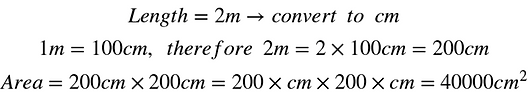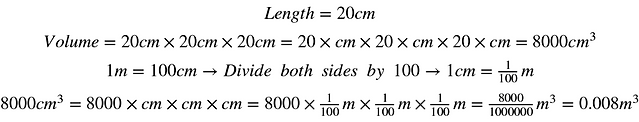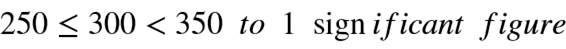#### 1.6 Units and Accuracy

When doing calculations, it is very important to be mindful of the units being used and the accuracy of the numbers being used; especially when doing calculations using data generated from scientific experiments. So if you plan on doing physics and chemistry pay particular attention to this topic.

## Units

A unit of measurement is a defined standard for measuring physical quantities. Mass, time, length, temperature, etc can be measured using specific units. Unit of mass can be kilograms or pounds or stones. Unit of temperature can Celsius or Fahrenheit or kelvin. As you can see there are different types of units available for measuring the same physical property.

If we measure one physical property using multiple types of units we cannot compare them together and carry out calculations. To prevent this we have systems of units in place which standardizes measurements. A system of units is a collection of units that are related to each other under certain rules.

The type of system of units used varies from country to country. However, the one system that is internationally accepted as the standard of measurement is the International System of Units or SI. It uses the metric system as its base.

In the metric system, units are expressed as multiples of a decimal pattern, meaning they increase or decrease in order of magnitude of 10. The chart below shows the prefixes you can use to express such measurements.As you can see, by using this scale it is possible to deal with very large or very small numbers in a manageable form. It makes calculations easier. It also makes it easier to understand the magnitude much more efficiently than having to count how many zeros there are in the number. Incorporating the metric scale into a unit makes the unit more versatile.

You can use the unit, meter, to measure something as small as an atom to something as large as a planet by using these prefixes. For example the length of our milky way galaxy is roughly 1000 Em, which is exameters. That is 1,000,000,000,000,000,000,000 m. The radius of a neutron is 0.8 fm, which is femtometers. That is 0.0000000000000008 m. It is much easier to use Em or fm rather than having to count how many digits there are every time you read or write the value.

Even though using units with prefixes makes calculation more efficient and easier, you have to be extra careful to make sure the units are uniform; or else your answers will be completely wrong. Let's look at how units can affect our calculations:

Example 1

There is a square whose sides are 2 m in length. Calculate the area. Express it in both m and cm.

# 2 m

So, we know that the formula for area is:

Area = length x width

Then let's check if all the dimensions are in the same units. In this case we have a square so all the sides are equal and the units are the same.

The steps are shown below for both m and cm:As you can see changing the units causes a big change in the magnitude as well. To calculate area in cm we had to first convert the length that was in meters to centimeters. You may be wondering if it is possible to convert the area that we already calculated in meters to centimeters without having to convert the length to centimeters.

It is possible but you have to be careful when using this method.

So we know that 1 m = 100 cm. We also know the area is 4m^2 so all you have to do is convert that m^2 to cm^2 without doing anything to the 4:Let's look at another example:

Example 2

This time there is a cube whose sides are 20 cm in length. Calculate the volume. Express it in both m and cm.

The formula for volume is :

Volume = length x width x height

Once again we have to make sure all dimensions are in the same units. This time since we are given the sides in cm let's find the volume using that unit first.To find volume in meters convert the length to m by dividing by 100 as shown below:As shown in Example 1, we can also directly convert the calculated volume to meters.Let's use the same method and calculate volume in millimeters:Let's do a couple problems with units of time:

Units of time can be confusing because you have to convert between days, hours, minutes and seconds. The conversion from seconds to minutes or minutes to hours, etc is not metric ; therefore making careless mistakes is likely. Before proceeding let's look at the conversions:It is important to note that just like the SI unit of length is meter (m), the SI unit of time is seconds (s). Therefore, when you are using the metric prefixes for time only use it with the seconds. You don't usually say micro-minutes or mega-days. Instead 31,536,000s can be expressed as 31.536 Ms (mega seconds).

Example 3

Your computer starts updating at 20.45 on Saturday. It completes the process the following day, Sunday, at 7.15. How long did your computer take to update?

One way to solve this is to break the time into segments. We know that Saturday is over by midnight, 24.00, so we should first calculate the time between 20.45 and 24.00. It takes 15 minutes for 20.45 to turn into 21.00. Then it takes 24 - 21 = 3 hours for 21.00 to turn to 24.00. Therefore the time spent on the Saturday portion is 3 hours and 15 minutes.

Next we find the time between 00.00 to 7.15 on Sunday. Why do we use 00.00 instead of 24.00? 24.00 is used when showing the end of a day and 00.00 is used when showing the start of a day. Since Saturday has ended we use 24.00 for Saturday and since Sunday has just started we use 00.00 for Sunday.

So it takes 00.00 - 7.00 = 7 hours for 00.00 to turn into 7.00 and then it takes another 15 minutes for 7.00 to turn to 7.15. Therefore the total time it takes is 3 hours and 15 minutes + 7 hours and 15 minutes = 10 hours and 30 minutes. It has taken your computer 10 hours and 30 minutes to complete its update.

## Accuracy

When measuring something, the magnitude that you get is not a 100% accurate. It is accurate only up to a certain degree. You can never really know what the true value of a measurement is because of uncertainity. As a result there is always a maximum value and a minimum value for any measurement you make. Your true value, which is unknown actually lies within this range.

Upper Bound and Lower Bound

If you are using a professionally calibrated measuring tool then this range will be very small, meaning your measurements fluctuate by a very small amount. However, if you are using a measuring tool that is not properly calibrated then the range of your measurements will be larger. This range of upper bound and lower bound helps you determine the accuracy of your calculation.

After you take a measurement sometimes it can be rounded off so that it is much easier to work with. By rounding it off you are generating a possible upper bound value and a lower bound value. Let's look at an example to understand how to calculate these bounds:

Example 4

Let's assume that you and your chemistry lab partner crystallize a solution. Your friends weighs the mass of the left over solid while you clean up. He tells you that the mass is 2.5 g rounded to 1 decimal place. What could the actual value have been before it was rounded to 1 decimal place?

To solve this you need to first understand that a value that has been rounded to the nearest unit would have had an actual value that was up to half a unit larger or half a unit smaller than the rounded value.

Let's use this to solve the problem. Your friend's measurement has be rounded to 1 d.p. Since a single unit of 1 d.p is 0.1, half of that unit is 0.05. For the maximum value or upper bound you would add this to the rounded value: 2.5 g + 0.05 = 2.55 g.

For the minimum value value or lower bound you substract this from the rounded value: 2.5 g - 0.05 = 2.45 g.

This makes sense because if you take the lower bound value,2.45, and round it off to 1 d.p you get 2.5.

But shouldn't the upper bound value be 2.54 rather that 2.55 because if you are rounding 2.55  to 1 d.p you get 2.6 g not 2.5 g. Then how can we say that 2.55 g is the upper bound value of 2.5.

2.54 is not the upper bound because 2.54 can also be written as 2.549 and it will still round to 2.5 when rounded to 1 d.p. 2.54 can also be written as 2.549999 or 2.54999999999999. As you can see it keeps going forever; since we don't want to write 2.54999 with an infinite number of 9s the correct value is 2.55.

Example 5

calculate the upper and lower bounds for the following values:

a) 8 cm rounded to the nearest cm

b) 5000 m to the nearest 1 significant figure

c) 300 to the nearest 1 s.f , 2 s.f and 3 s.f

d) 53.5 kg to the nearest tenth of a kg

a) Half a unit of a cm is 1 cm / 2 = 0.5

Therefore upper bound is 8 + 0. 5 = 8.5 and lower bound is 8 - 0. 5 = 7.5.b) Calculating upper and lower bounds to the nearest significant figures can be a bit confusing.

Rounding the upper bound down to 1 s.f should give you 5000. So you look at the digit following the 1 s.f. Therefore the upper bound must be 5500.

Rounding the lower bound up to 1 s.f should give you 5000. Therefore lower bound should be 4500.c) Rounding down upper bound to 1 s.f should give you 300. Therefore upper bound is 350.

Rounding up lower bound to 1.sf should give you 300. Therefore lower bound is 250.Rounding down upper bound to 2 s.f should give you 300. Therefore upper bound is 305

Rounding up lower bound to 2 s.f should give you 300. Therefore lower bound is 295.Rounding down upper bound to 3 s.f should give you 300. Therefore upper bound is 300.5

Rounding up lower bound to 3 s.f should give you 300. Therefore lower bound is 299.5d) Tenth is the first digit to the left of the decimal point which in this case is 5. This means rounding the upper bound and lower bound must give you 53.5. Therefore upper bound is 53.55 and lower bound is 53.45.Calculating upper bounds and lower bounds can be tricky sometimes, so always be careful and double check your answers to see if they will round to give you the rounded value. Practicing a lot of these types of questions will help you work out the bounds much faster and without making careless mistakes.